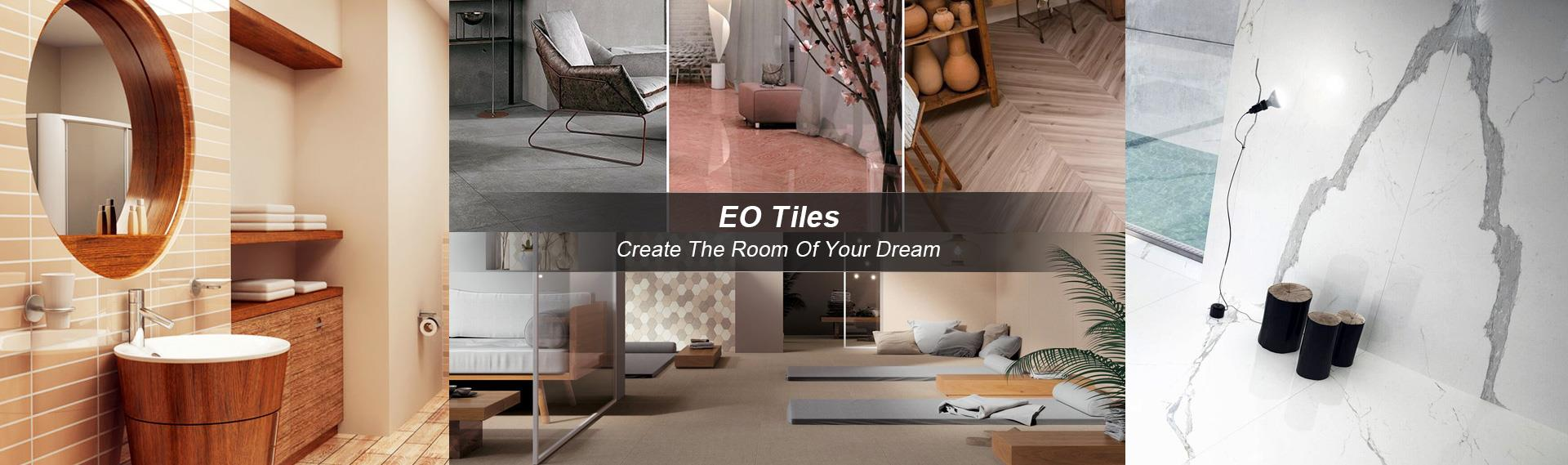Home » Knowledge » Content

# How to calculate the number of tiles accurately?

- Mar 26, 2018-

Buy tiles do the accurate calculation, that in the end there is no quick calculation of tile number method? Of course, a number of ceramic tiles is calculated according to the area, the second is calculated in accordance with the length of the waist piece.

1, according to area calculation of tiles number: the number of pieces = tiles paving area (square meter) (the width of a single ceramic tile with a single tile). If the decimal calculation results, after the decimal point "is a general method to calculate the loss should also be rounded, plus 3 or so.

2. The number of pieces of the waist line according to the length: the number of the total slice = the length of the total length of the waist line. For example: the length of the waist line is 10 meters, the size of the selected waist line is 80mm 3mm brick. After the decimal point calculation method commonly used to rounding, ready to buy 1-2 pieces a loss.